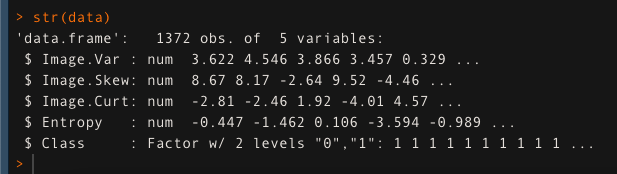Shivam Sharma — May 2, 2021

This article was published as a part of the Data Science Blogathon.

The Random Forest algorithm is undoubtedly one of the most popular algorithms among data scientists. It performs very well in both classification and regression problems. Random Forest is known as an ensemble technique for it is a collection of multiple decision trees.

### What was the main objective of using the multiple decision trees?

Using a single decision tree has several drawbacks. When we use a single decision tree to solve a problem statement, we encounter a situation of low bias and high variance. That is, the tree will capture all of the information about the training data as well as the noise. As a result, the model developed using the decision tree algorithm will perform nicely with training data but will perform poorly when evaluated on testing data (unknown data). Overfitting is the condition of having low bias and high variance.

Remember: –

Decision Tree—————> Overfitting————–> low bias high variance

Random Forest uses multiple decision trees to avoid this issue present in the decision tree algorithm.

### But, how does Random Forest address the problem of overfitting?

The Random Forest algorithm does not use all of the training data when training the model, as seen in the diagram below. Instead, it performs rows and column sampling with repetition. This means that each tree can only be trained with a limited number of rows and columns with data repetition. In the following diagram, training data 1 is used to train decision tree 1, and training data n is used to train decision tree n. However, since each tree is created to its full depth and has the property of overfitting, how do we avoid this problem?

Since the algorithm isn’t reliant on a particular decision tree’s outcome. It will first get the results from all of the decision trees, and then give the final result based on the problem statement type. For instance; if the problem statement type is classification, it would use majority voting. suppose we are classifying “yes” and “no” with 10 trees, if 6 trees are classifying “yes” and 4 are classifying “no”, the final answer will be “yes” using majority voting. What if our output is a continuous variable? In that case, the final result would be either the mean or the median of all trees’ output.

Classification Problem -> Majority Voting

Regression Problem -> Mean / Median

Here,

The model performs row sampling. However, feature sampling must be done according to the problem statement type.

• If the problem statement type is “classification”.

The total number of random features/ columns selected = p^½ or the square root of p,

where p is the total number of independent attributes/ features present in the data.

• If the problem statement type is “regression”.

The total number of random columns selected = p/3.

### Random Forest avoids overfitting with:-

1) Performing row and feature sampling.

2) Connecting all the decision trees parallelly.

### Why it is known as the Bootstrap Aggregation technique?

Random Forest is a type of ensemble technique, also known as bootstrap aggregation or bagging.

The process of sampling different rows and features from training data with repetition to construct each decision tree model is known as bootstrapping, as shown in the following diagram.

Aggregation is the process of taking all of the outcomes from each decision tree and combining them to produce a final outcome using either majority votes or average values, depending on the problem statement type.

## Random Forest using R

```library(caTools)

library(randomForest)```

We require to install ‘caTools’ and ‘randomForest’ libraries and activate them using the library() function

We have used the data set of banknote authentication and stored it in the ‘data’ variable. we will check the structure of data using the str() function.

str(data)Now we will split our data into train and test parts. 80% for training and 20 % for testing the model.

set.seed(123)
split <- sample.split(data,SplitRatio = 0.8)
train <- subset(data,split==T)
test <- subset(data,split==F)

After splitting the data, we will build our model using the randomForest() function. Here ‘ntree’ is the hyperparameter. which is required to be tuned. In this case, it is selected as 500.

random_model <- randomForest(Class~., data=train, mtry=2, ntree=500)

Predicting the model’s accuracy on the test data using the predict() function.

eval <- predict(random_model,test)

Evaluate the model’s accuracy using the confusion matrix

confusionMatrix(table(eval,test\$Class))

The model is giving an accuracy of 98.91% on the test data. This ensures that Random Forest is doing a fantastic job.

Thank You,

Shivam Sharma.

Phone: +91 7974280920

E-Mail: [email protected]

The media shown in this article are not owned by Analytics Vidhya and is used at the Author’s discretion.